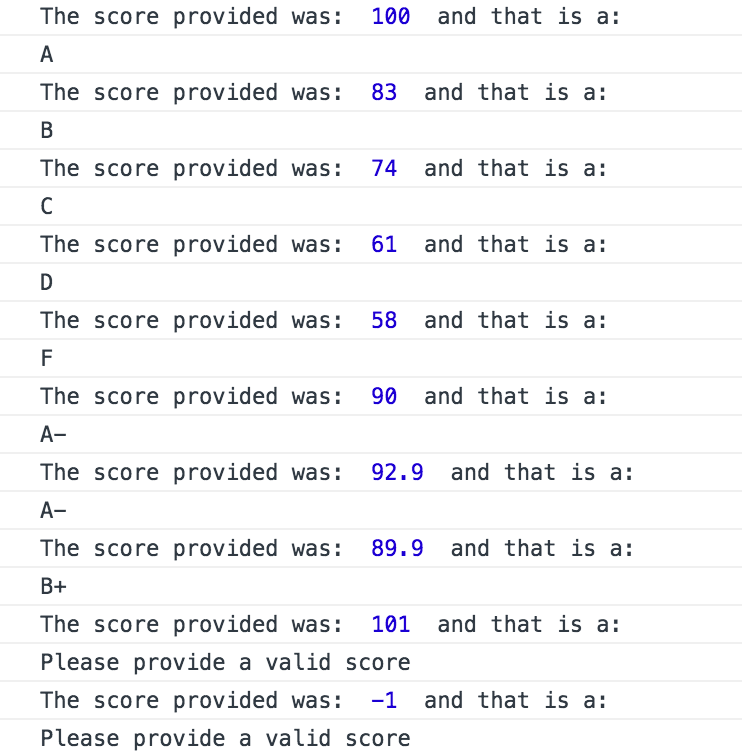# Assignment - Letter Grade Calculator

The goal of this excercise is to write a function to take a number and turn it into a letter grade. This will be a good practice of using >, <, >=, and <=. Below is the the example function called getLetterGradeFromNumber(). Use that function to convert the numbers to letter grades. Below the function are a few text inputs to make sure you handle each grade case. The example inputs and expected outputs are provided.

This is the example grading scale to implement. For instance, a score of 87 would yield a B+. A score of 79.9 would yield a C.

If a score above 100 or below 0 is provided output: 'Please provide a valid score'